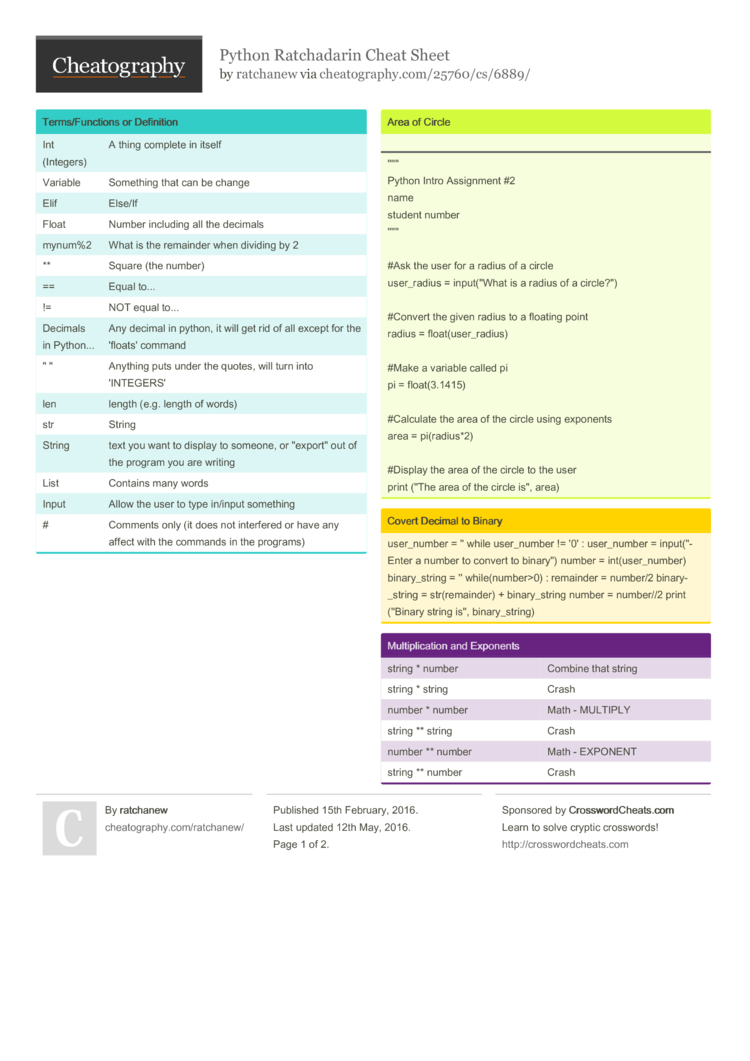# Python Ratchadarin Cheat Sheet by ratchanew

Include all the functions, vocabulary and other important concepts you have learned in Python so far.

### Term­s/F­unc­tions or Defini­tion

 Int (Integers) A thing complete in itself Variable Something that can be change Elif Else/If Float Number including all the decimals mynum%2 What is the remainder when dividing by 2 ** Square (the number) == Equal to... != NOT equal to... Decimals in Python... Any decimal in python, it will get rid of all except for the 'floats' command " " Anything puts under the quotes, will turn into 'INTEGERS' len length (e.g. length of words) str String String text you want to display to someone, or "­exp­ort­" out of the program you are writing List Contains many words Input Allow the user to type in/input something # Comments only (it does not interfered or have any affect with the commands in the programs)

### Area of Circle

"­"­"
Python Intro Assignment #2
name
student number
"­"­"

#Convert the given radius to a floating point

#Make a variable called pi
pi = float(­3.1415)

#Calculate the area of the circle using exponents

#Display the area of the circle to the user
print ("The area of the circle is", area)

### Volcabuary

 String A list of character such as number, letter and symbols ("Under quotes­" Variable Hold a value and can be change Integer Number Whole number (Can be positive or negative) Floating Point The number with decimals Syntax Gramma­r/S­tru­cture of Language Modulo Find the reminder Boolean True/False

### Countdown Machine

user_n­umber = input(­"What number do you want to count down? ")
number = int(us­er_­number)
countd­own­_string = ' '

while number > 0:
countd­own­_number = countd­own­_string + str(nu­mber) + " "
number = number - 1
#print­(nu­mber)

print (count­dow­n_s­tring)

### Covert Decimal to Binary

 user_n­umber = '' while user_n­umber != '0' : user_n­umber = input(­"­Enter a number to convert to binary­") number = int(us­er_­number) binary­_string = '' while(­num­ber­>0) : remainder = number/2 binary­_string = str(re­mai­nder) + binary­_string number = number//2 print ("Binary string is", binary­_st­ring)

### Multip­lic­ation and Exponents

 string * number Combine that string string * string Crash number * number Math - MULTIPLY string ** string Crash number ** number Math - EXPONENT string ** number Crash1 Page

PDF (recommended)